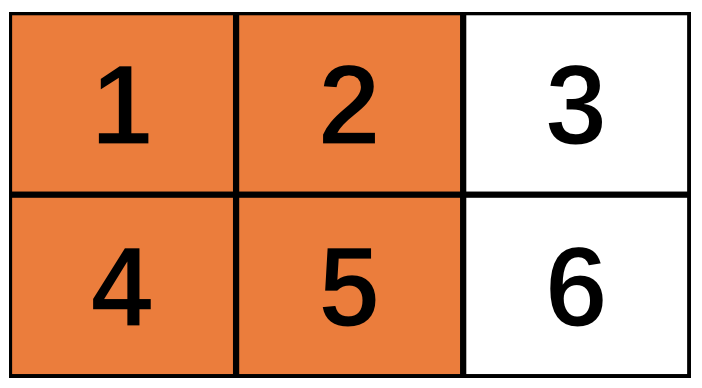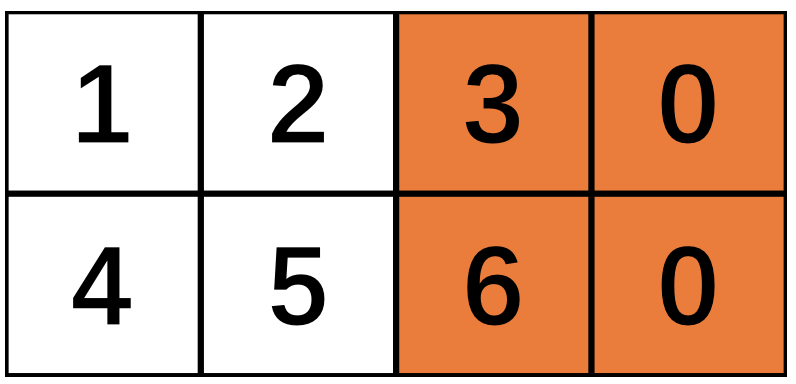def conv2d(input, filter, strides, padding, use_cudnn_on_gpu=None,
data_format=None, name=None)


padding: A string from: "SAME", "VALID".
The type of padding algorithm to use.


import tensorflow as tf

x = tf.constant([[1., 2., 3.],
[4., 5., 6.]])
x = tf.reshape(x, [1, 2, 3, 1])  # give a shape accepted by tf.nn.max_pool

valid_pad = tf.nn.max_pool(x, [1, 2, 2, 1], [1, 2, 2, 1], padding='VALID')
same_pad = tf.nn.max_pool(x, [1, 2, 2, 1], [1, 2, 2, 1], padding='SAME')



(1, 1, 1, 1)
(1, 1, 2, 1)If padding == "SAME":
output_spatial_shape[i] = ceil(input_spatial_shape[i] / strides[i])

output_spatial_shape[i] =
ceil((input_spatial_shape[i] -
(spatial_filter_shape[i]-1) * dilation_rate[i])
/ strides[i]).


$dilation\_rate$为一个可选的参数，默认为1，这里我们先不管。

$new\_height = new\_width =\lceil \frac{ (W – F + 1)} { S} \rceil$

$new\_height = new\_width =\lceil \frac{ W } { S}\rceil$